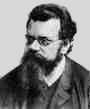Home

PHY 418: Statistical Mechanics I
Prof. S. Teitel stte@pas.rochester.edu ---- Spring 2012

## Problem Set 4

Due Monday, April 2, in lecture

• Problem 1 [15 points total]

Consider a system of particles in the grand canonical ensemble, with μ the chemical potential and z=exp(βμ) the fugacity. If < N> is the average number of particles, and <E> is the average total energy,

a) show that,

 1T ( ∂∂μ )T,V = 1kBT2 [ - ]

b) show that,

 ( ∂∂T )z,V = 1T ( ∂∂μ )T,V
Note, on the left hand side the derivative is taken at constant fugacity z, rather than constant chemical potential μ.

• Problem 2 [35 points total]

Consider a classical gas of very weakly interacting molecules of different species. There are Ni molecules of species number i, i = 1, 2, ..., m. The molecules of different species may have different masses and different internal degrees of freedom (such as vibrational or rotational modes), however you may assume that the molecules do not interact with each other. The molecules of a given species are indistiguishable from each other.

(a) Show that the canonical partition function for the gas has the form

Q = Q(1)Q(2)....Q(m)      where      Q(i) = [Q1(i)]Ni/Ni!
and Q1(i) is the single particle partition function for molecules of species i. [5 points]

(b) Using the result in (a), show that the total pressure of the gas is the sum of the pressures that each of the species of molecule would have on its own (total pressure is the sum of the "partial pressures"). Similarly show that the total entropy of the gas is the sum of the entropies that each species would have on its own. [5 points]

(c) By taking the appropriate derivative of the total Helmholtz free energy of the gas, compute the chemical potential µi of species i. Express your answer in terms of Q1(i). [5 points]

(d) Assume that the molecules are free (i.e. not in any external potential, except for their confinement to a box of volume V). Show that Q1(i) can be written as Q1(i) = Vq1(i)(T) where q1(i) depends only on temperature T. [5 points]

(e) Suppose that the species of molecules undergo the chemical reaction

a1A1 + ... + ajAj ↔ aj+1Aj+1 + ... + amAm
where ai is the number of molecules of species Ai (i = 1,...,j) that combine to create ak molecules of species Ak (k = j+1,...,m). As discussed in lecture, the equilibrium number of molecules of each species will be determined by the condition

a1µ1 + ... + ajµj = aj+1µj+1 + ... + amµm
(make sure you understand where this result comes from!). Use the above, and the results of the previous parts, to show that the equilibrium concentrations of the species are given by

([n1]a1 [n2]a2...[nj]aj )/([nj+1]aj+1...[nm]am) = K(T)
where ni is the concentration of species i, ni = Ni/V, and K(T) is a function of temperature only. Derive an expression for K(T) in terms of the q1(i)(T). The above result is known as the "law of mass action". [5 points]

(f) Consider the reaction

A + B ↔ C
Suppose that an energy Eo is released when A and B combine to form C, i.e. Eo is the binding energy of the molecule C when compared to its separated constituents A and B. Assume that A, B, and C are free point particles (i.e. no internal degrees of freedom are excited). By explicitly evaluating the single particle partition function of the three species, determine the function K(T). Remember, you must properly include the binding energy Eo of molecule C when you compute its partition function. [5 points]

(g) Suppose that initially there are equal concentrations of A and B, nA = nB = no, while the concentration of C is initially nC = 0. Find the resulting equilibrium concentrations of A, B and C, in terms of no and the function K(T). [5 points]